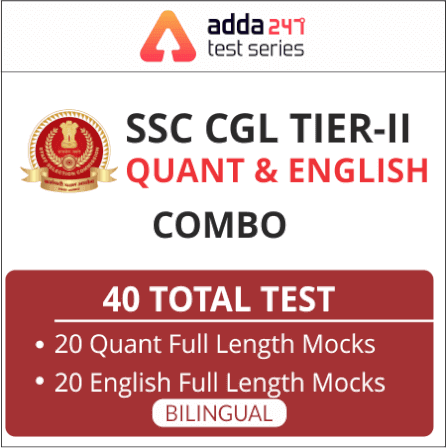Home Maths Notes Study Notes & Questions for SSC Exams: Trigonometry

# Study Notes & Questions for SSC Exams: TrigonometryMathematics is an equally important section for SSC CGL mains exam and has even more abundant importance in some other exams conducted by RRB & SSC. Generally, there are questions asked related to basic concepts and formulas of trigonometry.

To let you make the most of the Mathematics section, we are providing important facts related to Trigonometry. Also, RRB NTPC and State exams are nearby with bunches of posts for the interested candidates in which Mathematics is a major part. We have covered important notes and questions focusing on these prestigious exams. We wish you all the best of luck to come over the fear of Mathematics section.

Trigonometry

Important Results :-
• If tan α.tan β = 1 then α + β = 90°
• If sin α.sec β = 1 then α + β = 90°
• If cos α.cosec β = 1 then α + β = 90°
• If cot α.cot β = 1 then α + β = 90°
• If sin α.cosec β = 1 then α + β = 180°
• sin² θ + cos² θ = 1 , sin² θ = 1  – cos² θ & cos² θ = 1 – sin² θ
• sec² θ – tan² θ = 1 , sec² θ = 1 + tan² θ & tan² θ = sec² θ – 1
• cosec² θ – cot² θ = 1 , cosec² θ = 1 + cot² θ & cot² θ = cosec² θ – 1
• If sin² α + sin² β = 2 or sin α + sin β = 2 , then α = β = 90°
• If sin² + cos² β = 2 or sin α + cos β = 2 , then α = 90° & β = 0°
• if cos² α + cos² β = 2 or cos α + cos β = 2 ,then α = β = 0°
• sin² α + sin² β = 0 when α = β = 0
• sin² α + cos² β = 0 when α = 0 & β = 90°
• cos² α + cos² β = 0 when α = β = 90°

#### Click Here For Study Notes on Quantitative Aptitude For SSC CGLYou May Also Like To Read :×
OR

FORGOT?

×
OR